# The SSM Procedure

#### Delete-One Cross Validation and Structural Breaks

In addition to the interpolation of missing response values and the full-sample estimation of components in the model, the smoothing phase can also produce several useful diagnostic measures that can indicate outlying observations and breaks in the state evolution process. The treatment of additive outliers and structural breaks that is described in this section is based on De Jong and Penzer (1998).

##### Delete-One Cross Validation and the Additive Outlier Detection

Let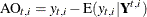denote the difference between the observed response value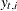and its estimate or prediction by using all the data except, which is denoted by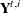. The smoothing phase of DKFS can generate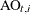(and its variance) at all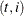. A large value ofsignifies that the observed response value () is unusual relative to the rest of the sample (according to the postulated model). Such values are called additive outliers. In the literature,are referred by a few different names. Sometimes they are called delete-one cross validation errors or simply prediction errors. In this chapter, these names are used interchangeably. Like the one-step-ahead residuals,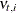, the prediction errors can be used in checking the adequacy of the model. The prediction errors are normally distributed; however, unlike, they are not serially uncorrelated.is set to missing whenis missing. The SSM procedure prints a summary table of extreme additive outliers by default. In addition, you can request the plotting of the standardized prediction errors, and they can be output to a data set.

The prediction error sum of squares (PRESS)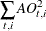can be a useful measure of fit to compare different models. It is also called the cross validation error sum of squares. An additional measure of fit based on the prediction errors is called the generalized cross validation error sum of squares (GCV). Denoting the variance ofby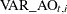, it is defined as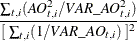You can request the printing of PRESS and GCV by specifying the PRESS option in the OUTPUT statement.

After inspecting the reported additive outliers, you can adjust the model to account for the effects of some of the extreme outlying observations. This can be done by including appropriate dummy variables in the observation equation.

##### Structural Breaks in the State Evolution

The additive outliers that are discussed in the preceding section are diagnostic measures associated with the measurement equation. The smoothing phase of DKFS can generate diagnostic measures that are also associated with the state equation.

For simplicity of notation and exposition, initially assume that the state equation has the following form: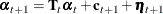That is, the state regression term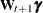is absent in the postulated model. Suppose that an unanticipated change of unknown size takes place in the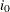th element of the state at time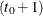. The model can then be adjusted to account for this change by including a suitable dummy regressor in the state equation as follows: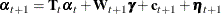Here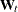is a sequence of m-dimensional column vectors such that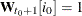and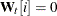for all other t and i. The estimate of the regression coefficient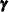provides information about the size of the unanticipated change in theth element of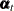at time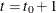. Similarly, an unanticipated change in a subsection ofat a timecan be estimated by using a set of appropriate dummies (the number of dummies equals the number of elements in the state subsection) in the state equation. The algorithm of De Jong and Penzer (1998) efficiently generates the estimates of such one-time changes in the state at all distinct time points in the sample in one smoothing pass. A statistically significant value ofat a time point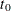indicates an unanticipated change in the relevant element (or the subsection) of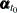. Note that the change associated with an additive outlier is temporary: the previous or the subsequent measurements are not affected. On the other hand, because of the evolutionary nature of the state equation, a one-time change in the state affects all the subsequent states, which in turn affect the subsequent observations. In this sense, a significant unanticipated change in the state is a structural break.

In the preceding discussion, the absence of the state regression variables in the postulated model was assumed only for notational simplicity. If the postulated model does contain some state regression variables, the dummy variable that is associated with the one-time state change is simply added to the existing set of state regression variables, and the interpretation of its regression coefficient as the measure of unanticipated change in the state remains unaffected.

In the SSM procedure, you can request the computation of significance statistics that are associated with one-time changes in the state subsections specified by using the STATE statement in addition to the state subsections that are associated with the components specified by using the TREND statements. This is done by using the CHECKBREAK option in these statements. In addition, you can request the computation of such statistics for the entire stateby using the MAXSHOCK option in the OUTPUT statement. The significance statistics can be computed for both elementwise change and subsectionwise change. The computation of subsectionwise change statistics can be computationally expensive for large subsections (an inversion of a
-dimensional matrix at each distinct time point in the sample is needed for the computation of significance statistics for a state subsection of size p). For an example of structural break analysis, see Example 27.8.